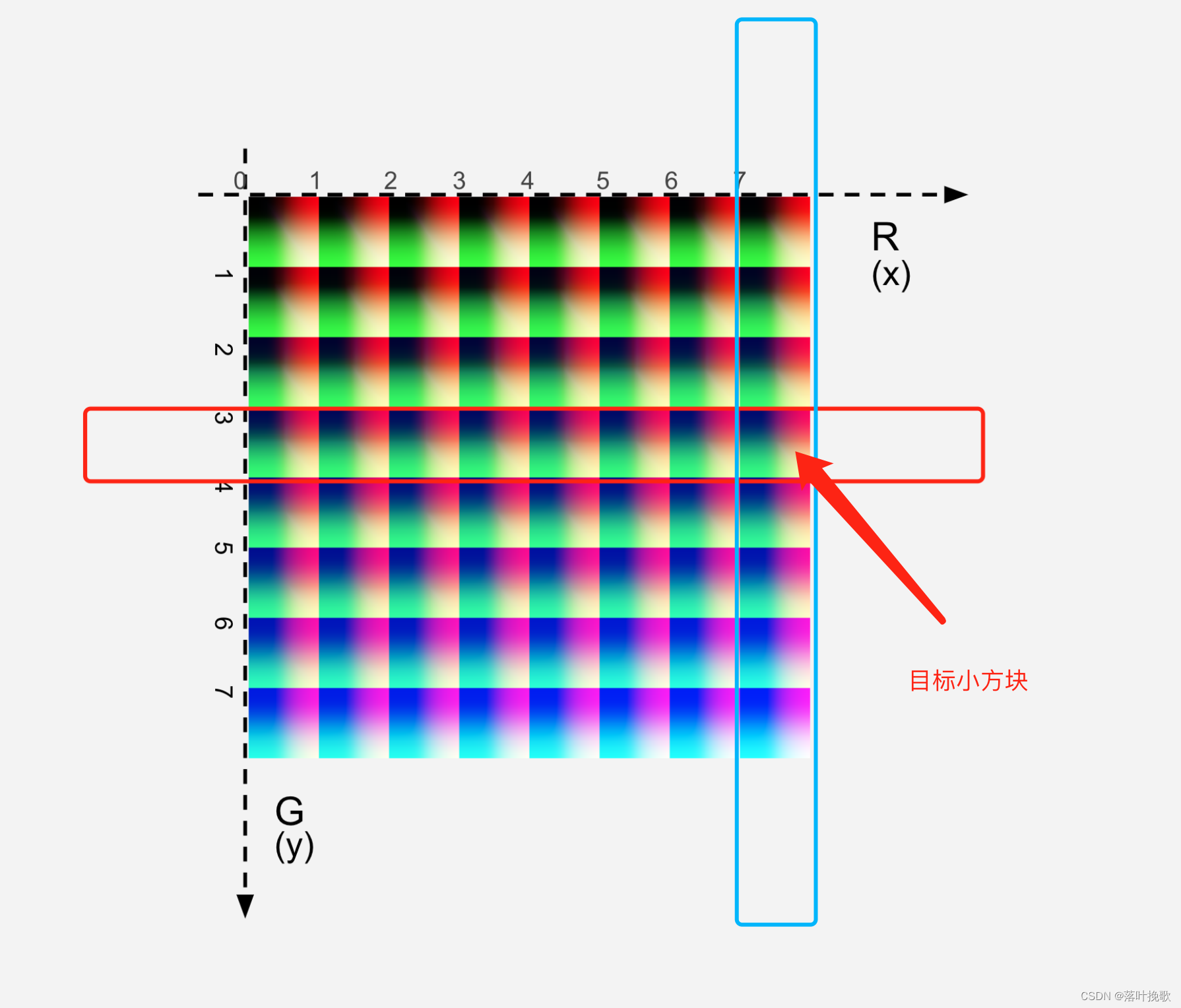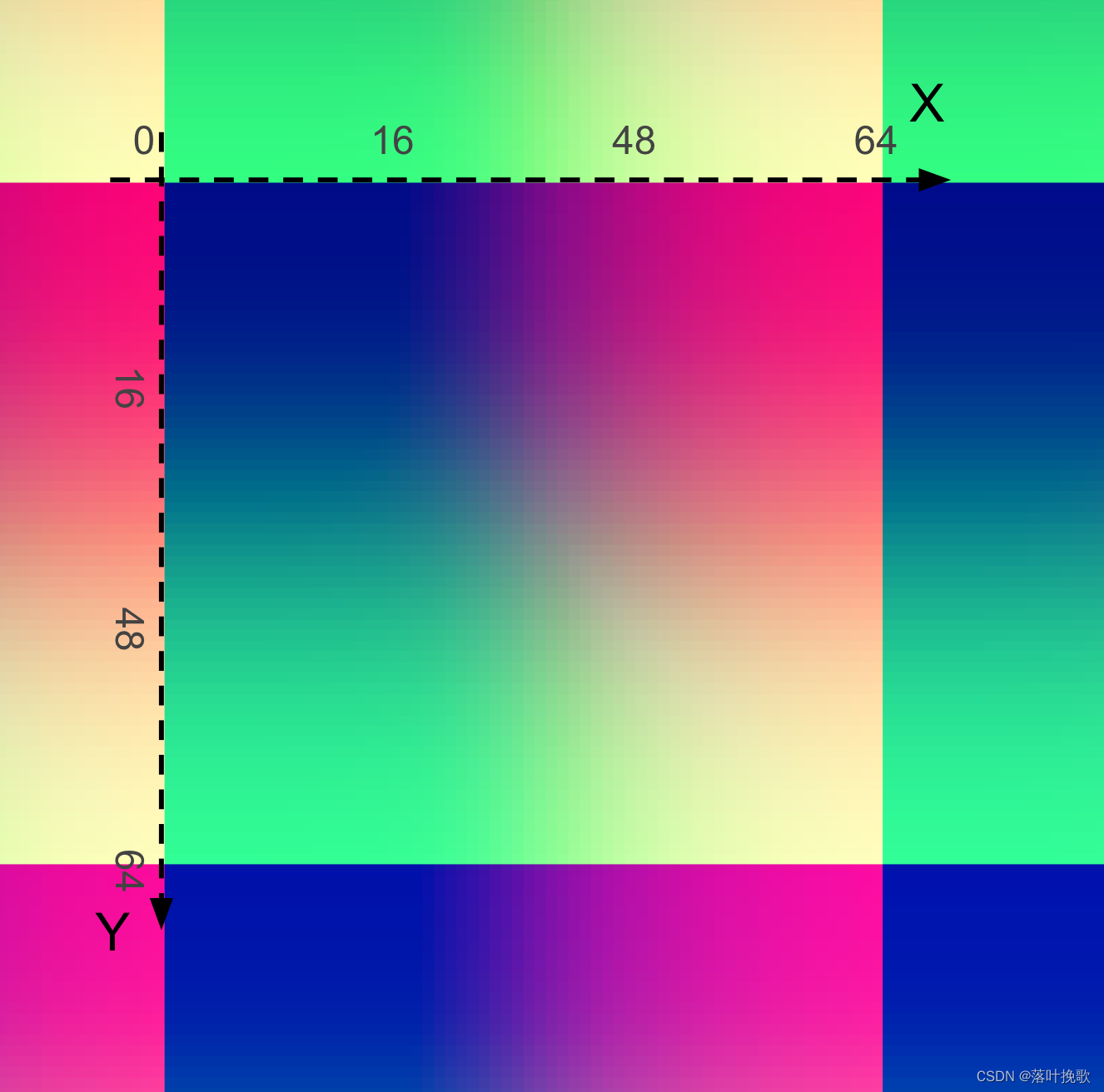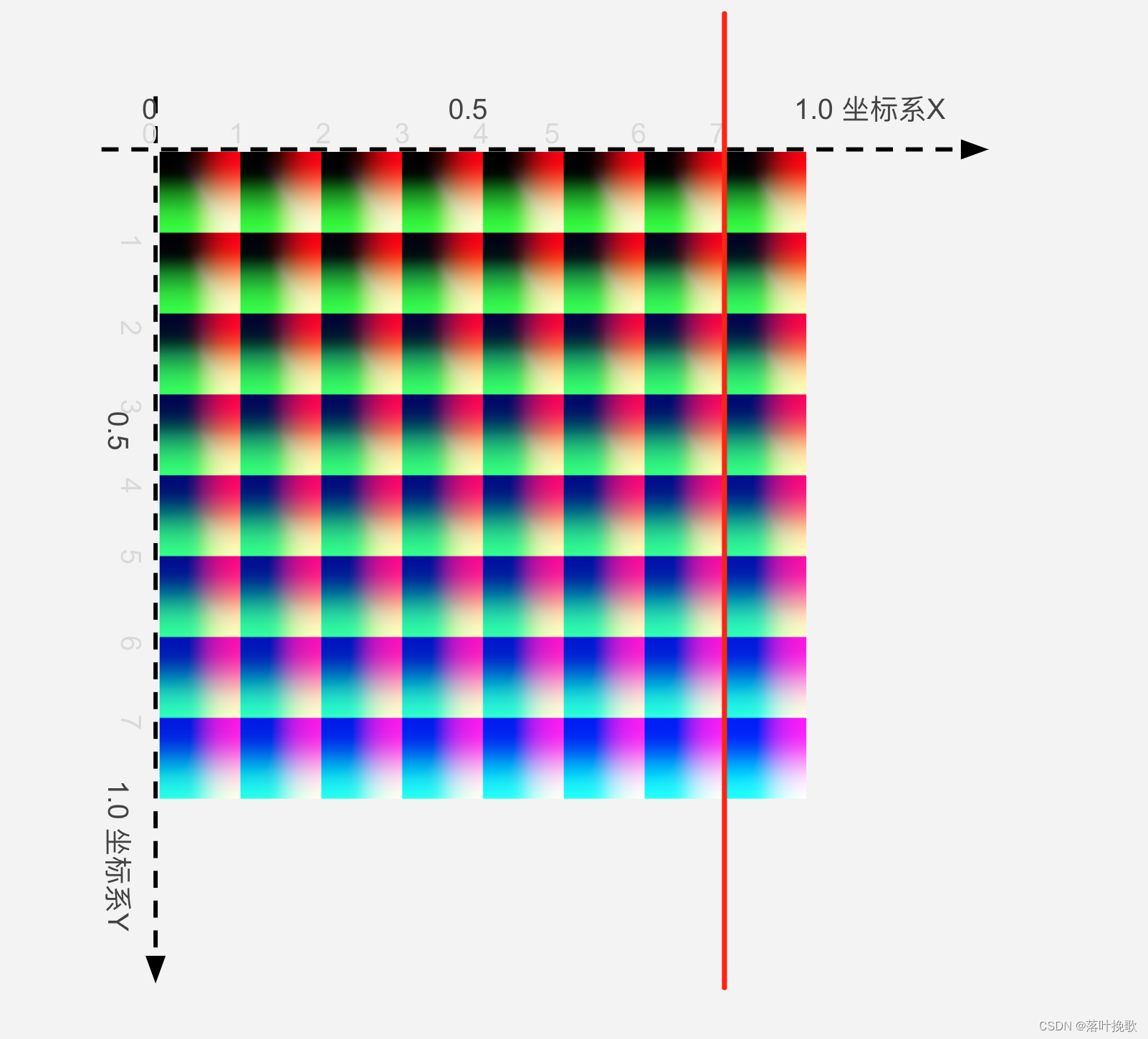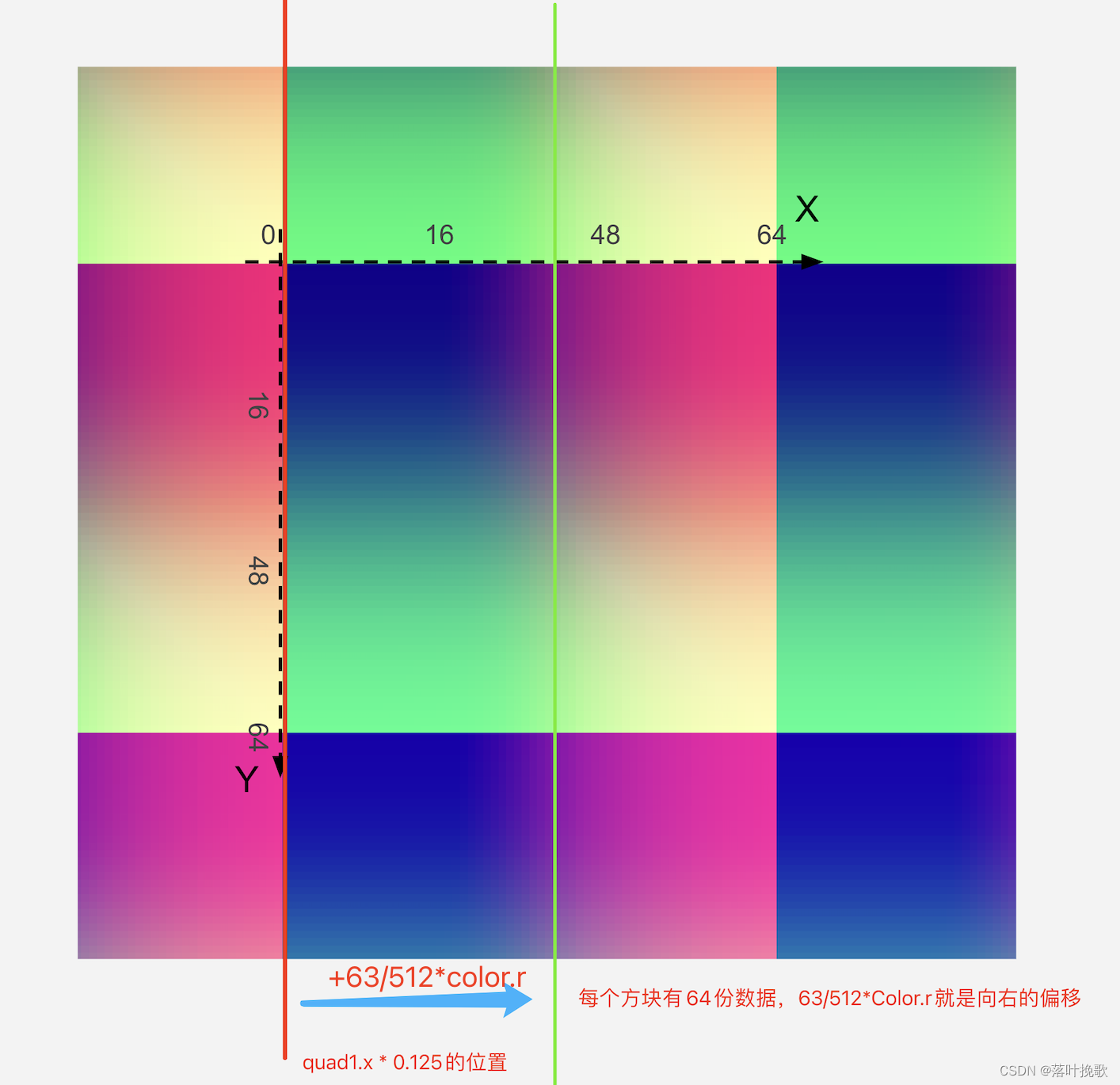textureColor = ver4(.0, .0, 0.5, 1.0)

## 查找颜色所在位置的小正方形

`textureColor.b * 63.0` = 31.5

quad1.y = floor(floor(blueColor) / 8.0) = 3，确定为小方块在纵坐标索引3，也就是第4行。`quad1.x = floor(blueColor) - (quad1.y * 8.0) = 31 - 24 = 7``quad2.y = floor(ceil(blueColor) / 8.0) = 4`

`quad2.x = ceil(blueColor) - (quad2.y * 8.0)= 0`

## 查找小正方形内的具体颜色`texPos1.x = (quad1.x * 0.125) + 0.5/512.0 + ((0.125 - 1.0/512.0) * textureColor.r)`